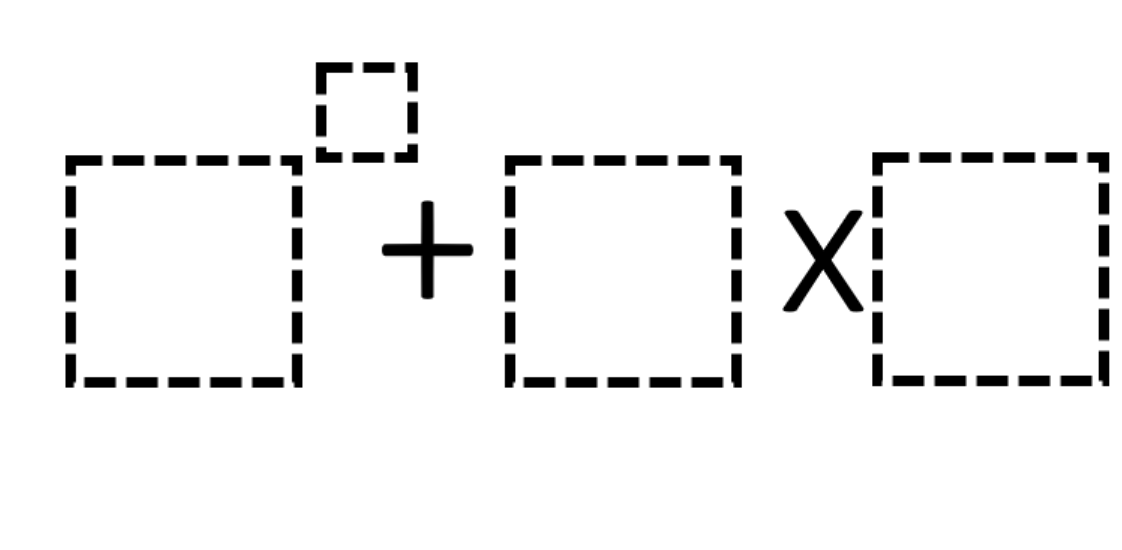# Order of Operations 6

Directions: Using the digits 1 to 5, at most one time each, place a digit in each box to create an expression with the largest possible value.### Hint

Where would you put your largest digit?

### Answer

4^5+2*3 or 4^5+3*2

Source: Matt Donahue

## Ratios 1

Directions: Using the digits 0 to 9 at most one time each, fill in the …

### 79 comments

1.my answer was:5 to the 4 power +4×2=1258

•I thought you could only use the numbers 1-5 one time.

•at the top it says “Using the digits 1 to 5, at most one time each”

•Sadalia Calderon

My answer was 4 to the power of 5 because I did 4^5+3*2

2.my answer was 5 to the 4 power +4×2=1258 too

•Remember… order of operations matter. Most teachers use GEMS or PEMDAS. In any case, you would need to multiply first before adding.

Example: 5 to the 4th power plus 4 times 2 would be…(exponents first)… 625 plus 4 times 2… (then multiply second)… 625 plus 8… (finish it)… 633.

Hope this helps!

•If you did pemdas it would be different because (5⁴+4)2 would then be (625+4)2 then (1250+8)=1258. But all of these won’t work because you can only use each number once and 4 is used twice in all of these.

3.5^4+3×2

4.2+(3×7+9)=(1-5)/(8×4)
2x(3/7-9)=(1+5)x(8+4)
2-(3x7x9)=(1-5)-(8/4)
22/(3-7/9)=(1×5)(8-4)
2x(3+7+9)=(1/5)(8×4)

5.2^3+4×1=12
1^2+3×5=16
5^3+4×3=137
4^4+5×2=266
5^5+1×2=3127
4^5+3×1=1027

•4^5+3*2

•You can only use 5 once

6.4^5+3×2=1030

7.4^5+2*3 or 4^5+3*2 is the answer I think.

8.4^5+2*3 or 4^5+3*2

9.5^4+3×2=1,256
4^3+5×2=138
4^5+3×1=1,027
3^5+2×4=980

10.4²+5×2 or 5²+2×4

11.5^4+4×2= 258

12.13.14.I got 2 over the power of 3 +5×4

15.It would be 5 to the power of 4 and the answer to that would be 625.

16.4^5+2*3

17.Barbie Cardenas

the answer will be 4^5+2*3 or it could be 4^5+3*2 any of these can be use as an answer.

18.2
4 +4×5=

16+ 20 = 36

19.2
3 +4×5= 9+ 20 =29

20.21.5^3+2*4 = 133

22.23.4^5 + 2*3 = 1030

24.4^5+2*3 or 4^5+3*2

25.4^5 + 5*3 = 1039

•My answer was 5 to the 4 power +4×2=1258 too

•You can’t use 4 more than one time according to the directions.

26.4^5+2*3 or 4^5+3*2

•4^5+3*2

27.I think it is 4^5+3*2

28.I believe it is 4 with an exponent of 5 plus 2 times 3

29.I think it is 4^5+2*3

30.31.4^5+2×3=1030

32.33.5^4+3*2 or 4^5+3*2

34.35.36.I don’t understand it also as my reflection it it was easy

37.38.5^4 + 4*3

39.4^5+2*3

40.41.42.My answer that I got was 4^5+2*3.

43.44.4 to the 5th power +3 X 2=2054

45.i was going to guess 5^4=3×2

46.4*5+3×2

47.48.49.50.51.5^4+3•2

52.4^5+3•2

53.Eliott Germain-Aubrey

4^5+3*2

54.My answer is 4^5+2×3

55.I got 4^5+2*3

56.I got 4^5+3*2

57.My answer was 4^5+2*3

58.My answer 4^5+3*2

59.My answer is 4^5+2*3=26

60.4^5+2*3=1030

61.4^5+2×3

62.Yes that was what i got too.

63.5/4+2×3

64.my answer was 4^5+2*3

65.4^5+3*2

66.I did (4^5*3)+2

•The answer to mine was 3074

67.4^5+3*2= 1030
1024+6=1030

68.